Potato chips and ANOVA in analytical chemistry, Part 1: Formatting data in JMP

Editor's Note: This post was written by @eric_cai, a chemist and statistician who also blogs at The Chemical Statistician. Follow Eric on Twitter @chemstateric.

Sample preparation is a very important part of measuring quantities of substances in analytical chemistry. One benefit of a good sample preparation scheme is the minimization of the cumulative uncertainty for the estimated quantity of interest. This two-part blog series will show how a basic statistical technique called analysis of variance (ANOVA) can assess the uncertainty that is introduced in a sample preparation scheme and offer insights on how it can be improved to minimize the cumulative uncertainty.

The first part of this series will introduce the problem and shape the data into a format that is ready for analysis. The second part of this series will use ANOVA to partition and compare the two sources of variation in a proposed sample preparation scheme.

Measuring Sodium in Potato Chips

A common ingredient in potato chips is table salt, or sodium chloride. Suppose that you want to measure the weight percentage of sodium in a bag of potato chips. Here is one possible scheme for drawing samples of chips out of this bag and preparing them for measurement. In this example, the quantity of interest – usually called the analyte in chemistry – is sodium.

• Randomly draw and weigh four chips from a bag.

• Grind each chip into a homogeneous paste.

• Dissolve each sample of paste in an Erlenmeyer flask of water.

• Draw three sub-samples (called aliquots) of equal volume from the homogenized sample each flask. Put each aliquot into a volumetric flask.

• Use an analytical instrument or technique to measure the weight percentage of sodium from each aliquot.

• Calculate the average of the 12 weight percentages from the 12 aliquots.

The following is a diagram that summarizes this scheme.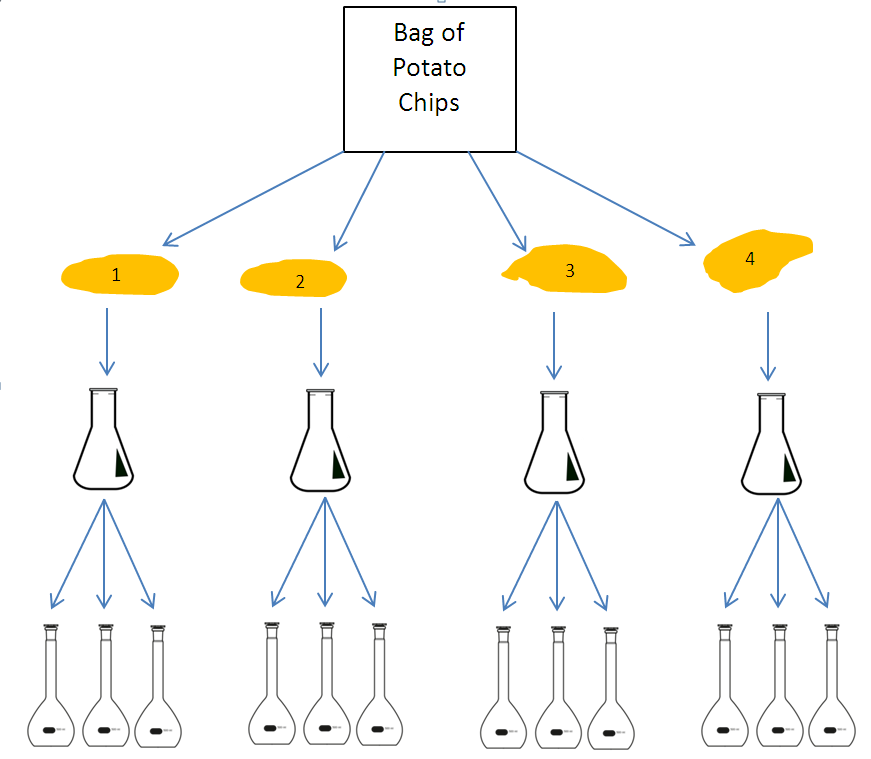Image sources: “Erlenmeyer flask” by Danilo Prudêncio Silva and "Volumetric flask" by Lucasbosch - Own work. Licensed under CC BY-SA 3.0 via Wikimedia Commons.

Here is a data set of measured weight percentages of sodium from the 12 aliquots; I obtained it from page 736 in chapter 29 of the 6th edition of “Quantitative Chemical Analysis” by Daniel Harris.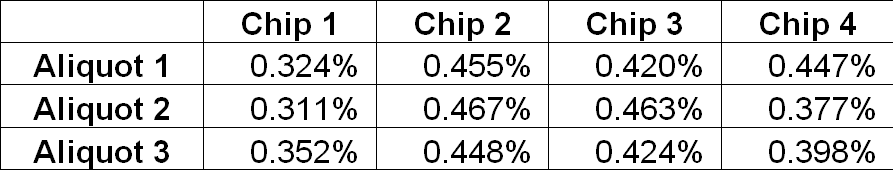Estimating the true weight percentage of sodium in this bag of potato chips can be done in a relatively straightforward manner – simply pick a good analytical technique, build a calibration curve, and use inverse prediction to obtain a point estimate and a confidence interval. However, this blog post will focus on the variation that is introduced throughout this sample preparation process and how it can be minimized. This process is critical to minimizing the cumulative uncertainty for the final measurements of the weight percentages.

Entering and Transforming the Data

Let’s enter the above data set into JMP.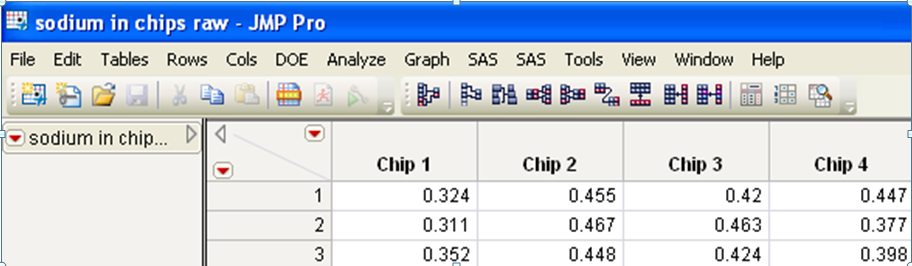If you prefer to show the first aliquot under Chip 3 as 0.420, you can change this in the Column Properties. Highlight all columns, and then choose Standardize Attributes under the Cols menu.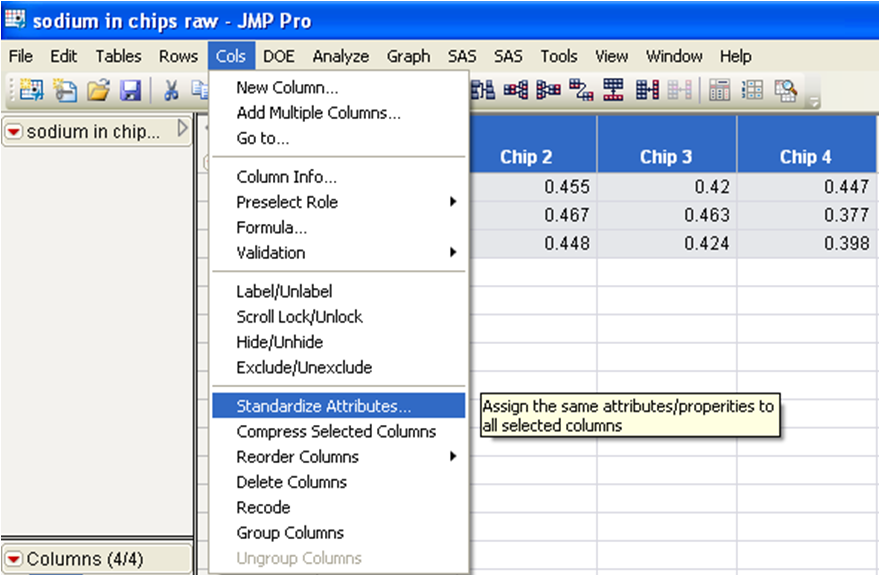In the Attributes drop-down list, choose Format. This activates the Format for modification.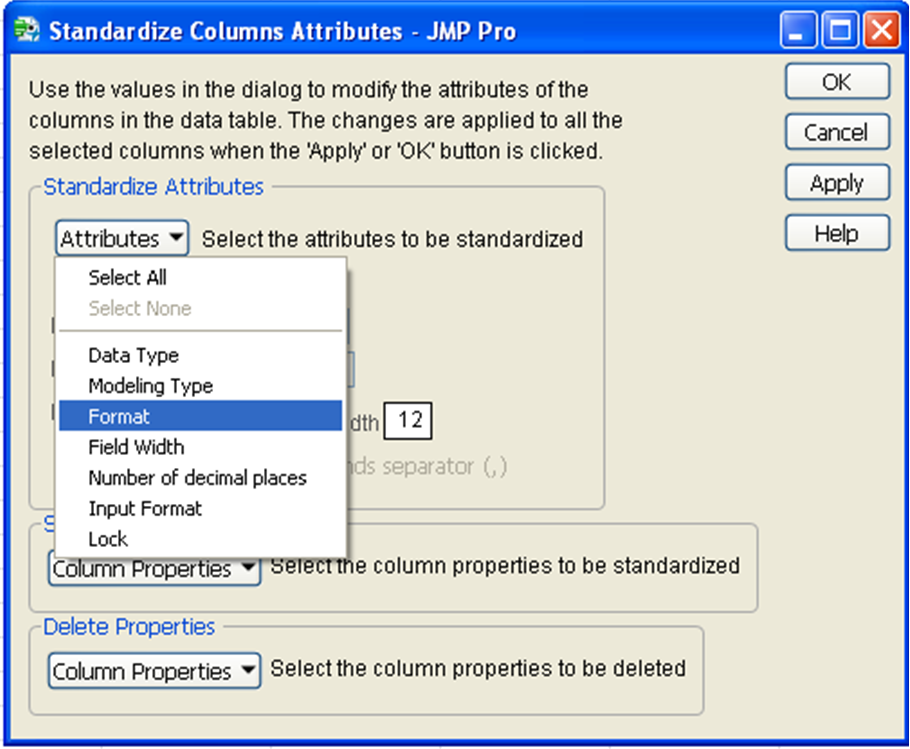Change the format from Best to Fixed Dec. In the newly available Dec field, change the value from 0 to 3.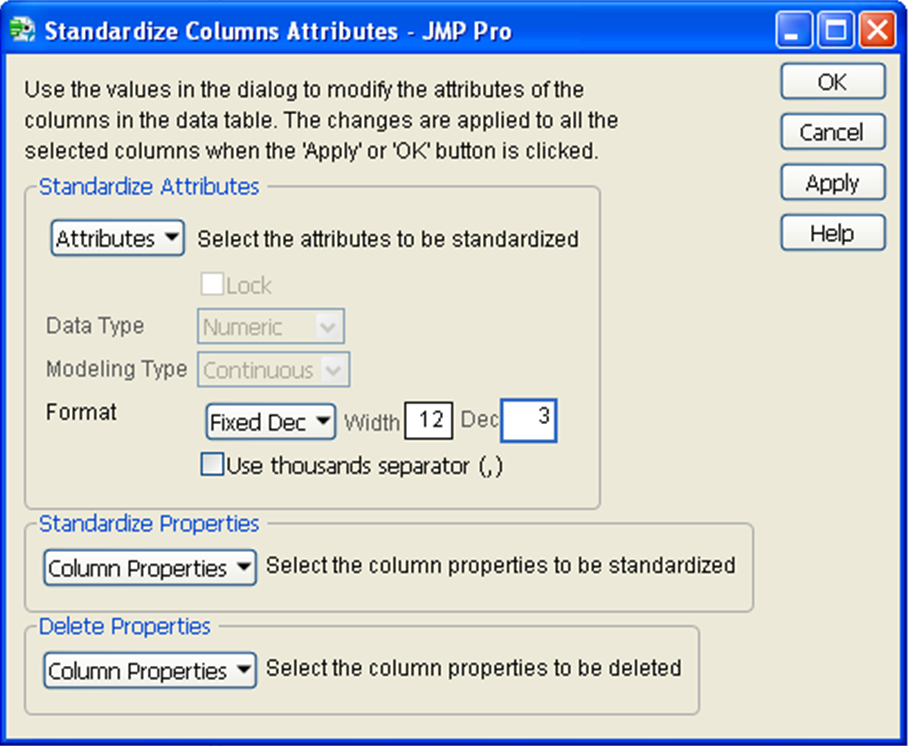Notice now that the first aliquot under Chip 3 now shows 0.420.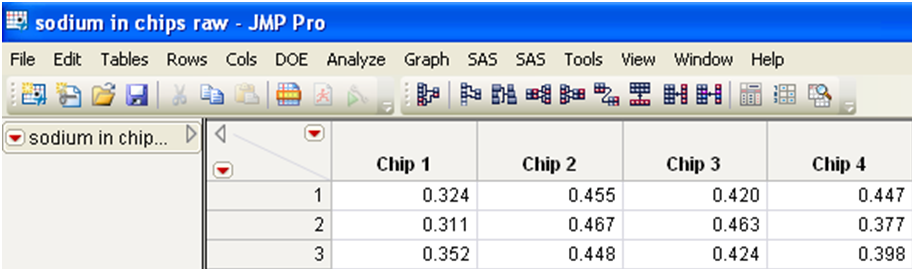As you will see later in this blog post, the layout of this data set is not ready for analysis in JMP. Instead, let’s stack this data set so that all data values are in one column, and another column indicates which chip each value came from. We will later use the Fit Y by X platform in JMP, and it requires the data to be structured in this stacked format.

Under the Table menu, choose Stack.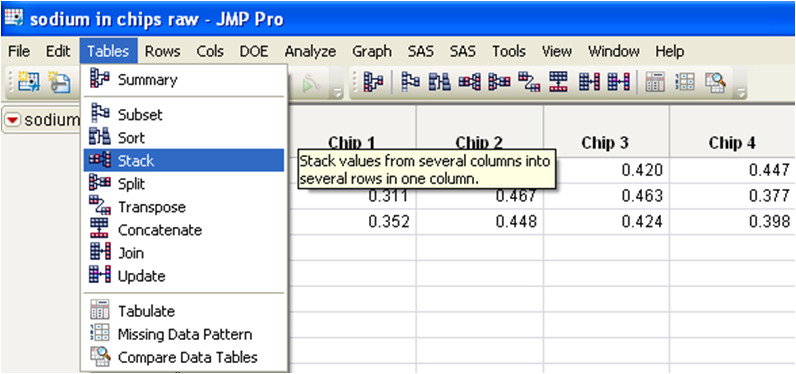Under Select Columns, choose all four chip columns, and then click Stack Columns. This will ensure that all four columns will be stacked. I have also entered the new names of the output table, the stacked data column, and the source label column.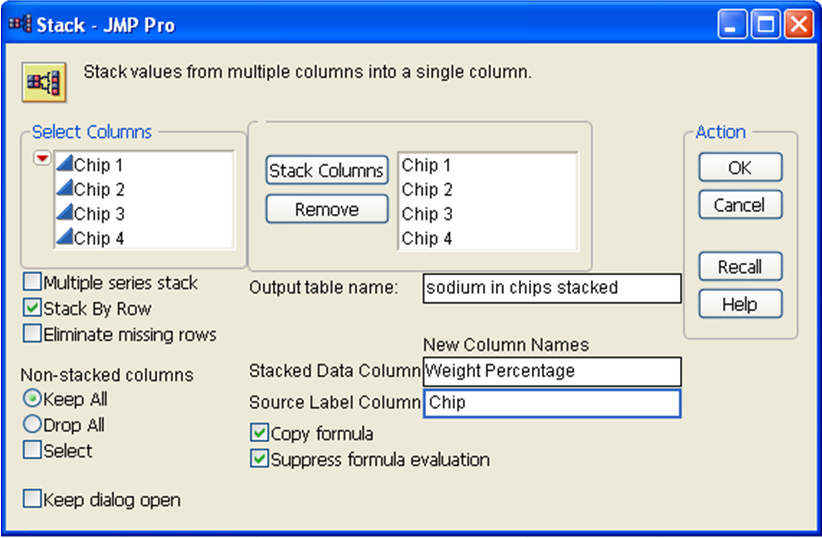Here is what the stacked data set looks like.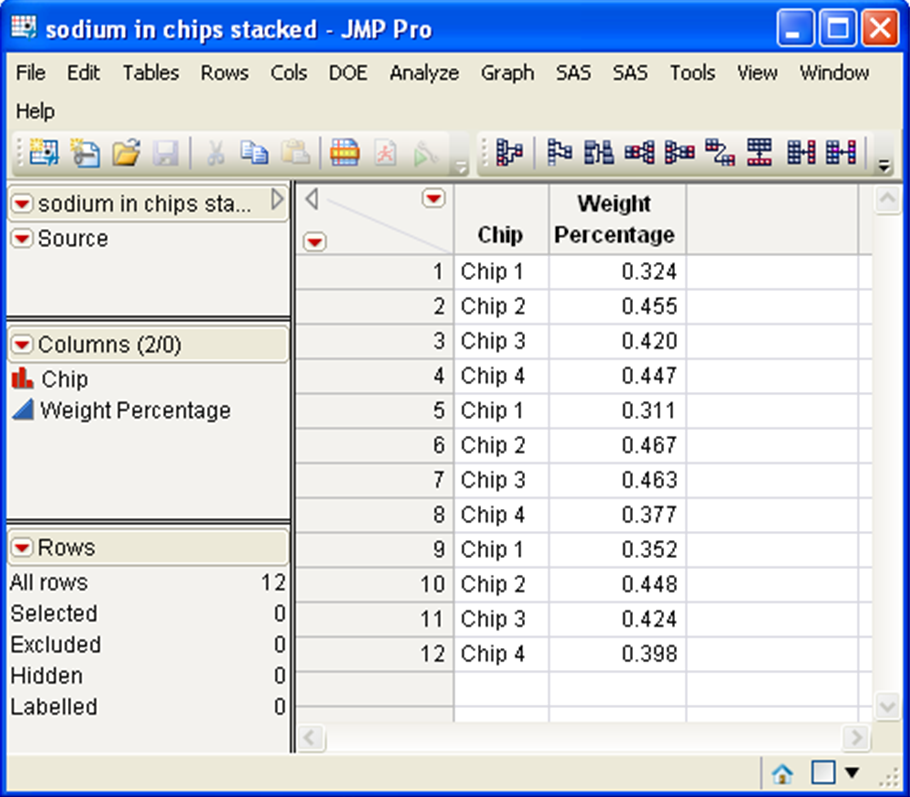The data are now ready for analysis! In the next blog post of this two-part series, I will use the Fit Y by X platform to visualize the data and analyze them using ANOVA.

I will conclude this series by interpreting the ANOVA results to answer our original question about comparing the variation in the two stages of our sample preparation scheme.

Stay tuned!

Reference

Harris, D. C. (2002). Quantitative chemical analysis (6th edition). Macmillan.

Article Labels

There are no labels assigned to this post.

Visitor

Shalin wrote:

The diagram explains itself well. Picture worth more than 1000 words after all.

Regards,

createlyStaff

Eric Cai wrote:

I'm glad that you liked it, Shalin! I worked really hard on all of my visualizations in this series of blog posts, and it's gratifying to know that they illustrate the concepts well! Thanks for reading!